# Flexible molecules

Jump to: navigation, search

Modelling of internal degrees of freedom, usual techniques:

## Bond distances

Atoms linked by a chemical bond (stretching) using the harmonic spring approximation: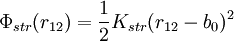$\Phi_{str} (r_{12}) = \frac{1}{2} K_{str} ( r_{12} - b_0 )^2$

However, this internal coordinates are very often kept constrained (fixed bond distances)

## Bond Angles

Bond sequence: 1-2-3:

Bond Angle: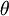$\left. \theta \right.$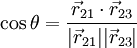$\cos \theta = \frac{ \vec{r}_{21} \cdot \vec{r}_{23} } {|\vec{r}_{21}| |\vec{r}_{23}|}$

Two typical forms are used to model the bending potential: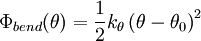$\Phi_{bend}(\theta) = \frac{1}{2} k_{\theta} \left( \theta - \theta_0 \right)^2$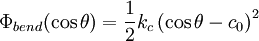$\Phi_{bend}(\cos \theta) = \frac{1}{2} k_{c} \left( \cos \theta - c_0 \right)^2$

## Dihedral angles. Internal Rotation

Bond sequence: 1-2-3-4 Dihedral angle (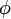$\left. \phi \right.$) definition:

Consider the following vectors:

•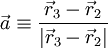$\vec{a} \equiv \frac{\vec{r}_3 -\vec{r}_2}{|\vec{r}_3 -\vec{r}_2|}$; Unit vector in the direction of the 2-3 bond
•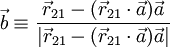$\vec{b} \equiv \frac{ \vec{r}_{21} - (\vec{r}_{21}\cdot \vec{a} ) \vec{a} } { |\vec{r}_{21} - (\vec{r}_{21}\cdot \vec{a} ) \vec{a} | }$; normalized component of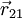$\vec{r}_{21}$ ortogonal to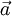$\vec{a}$
•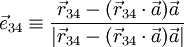$\vec{e}_{34} \equiv \frac{ \vec{r}_{34} - (\vec{r}_{34}\cdot \vec{a} ) \vec{a} } { |\vec{r}_{34} - (\vec{r}_{34}\cdot \vec{a} ) \vec{a} | }$; normalized component of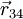$\vec{r}_{34}$ ortogonal to$\vec{a}$
•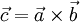$\vec{c} = \vec{a} \times \vec{b}$
•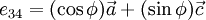$e_{34} = (\cos \phi) \vec{a} + (\sin \phi) \vec{c}$

For molecules with internal rotation degrees of freedom (e.g. n-alkanes), a torsional potential is usually modelled as:

•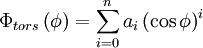$\Phi_{tors} \left(\phi\right) = \sum_{i=0}^n a_i \left( \cos \phi \right)^i$

or

•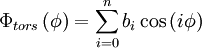$\Phi_{tors} \left(\phi\right) = \sum_{i=0}^n b_i \cos \left( i \phi \right)$

## Van der Waals intramolecular interactions

For pairs of atoms (or sites) which are separated by a certain number of chemical bonds:

Pair interactions similar to the typical intermolecular potentials are frequently used (e.g. Lennard-Jones potentials)